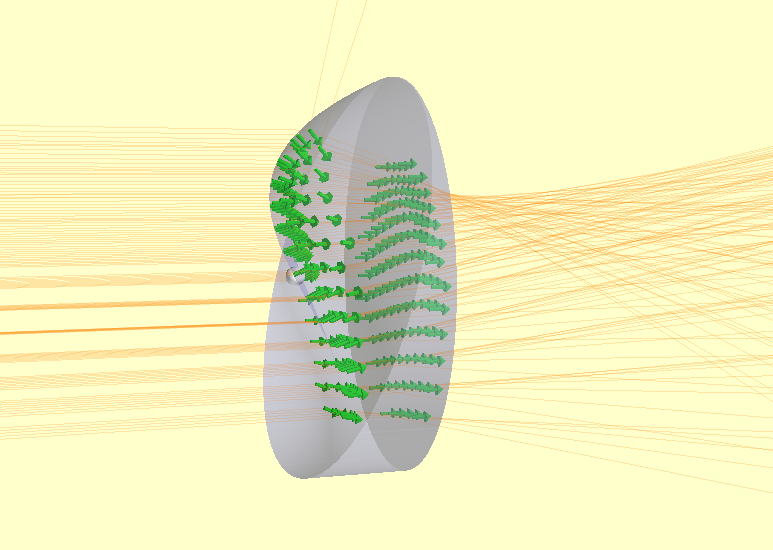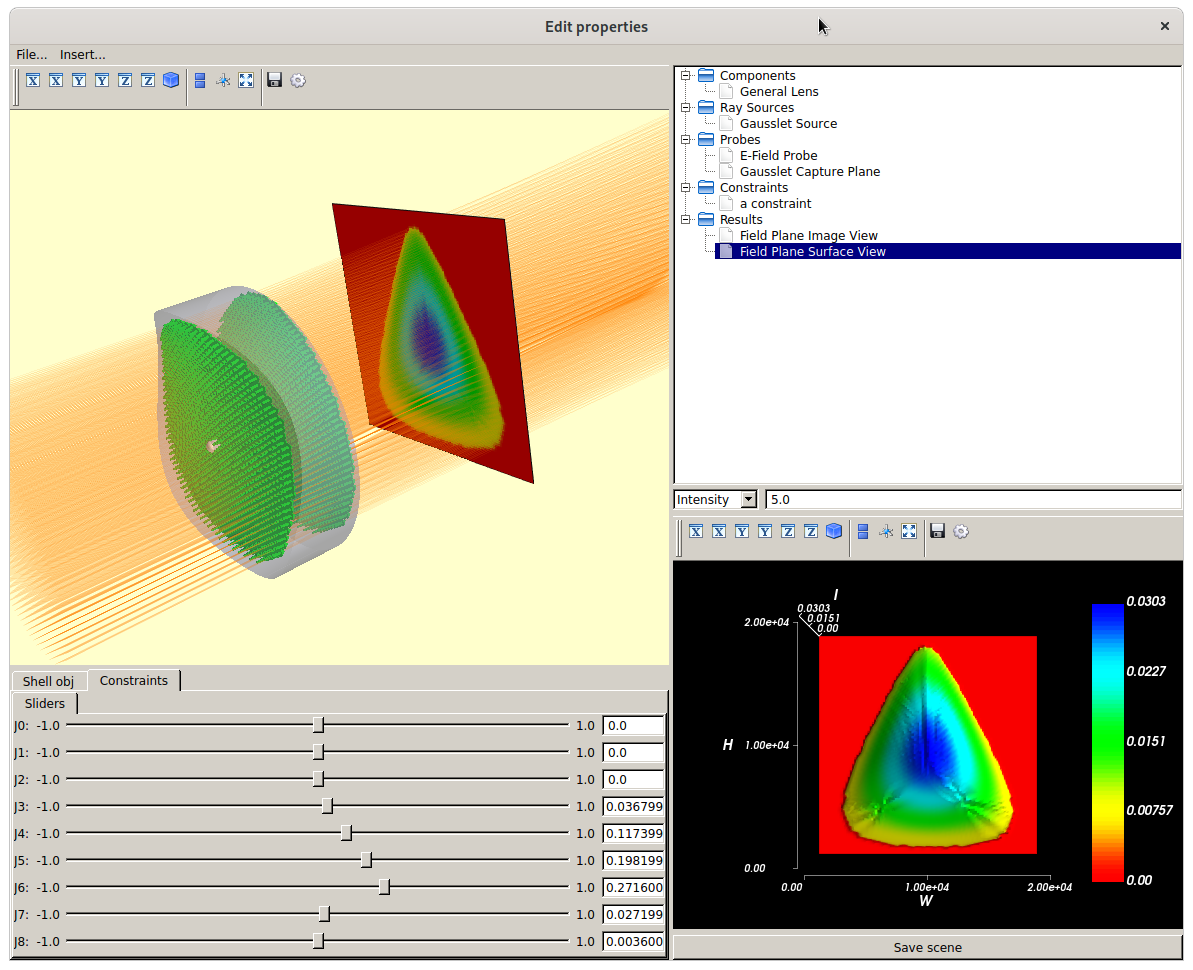Distortions¶

The raypier.distortions module contains objects representing distortions of a given face. The Distortion objects are intended to be used with the raypier.faces.DistortionFace class (part of the General Optic framework). Fundamentally, any 2D function can be implemented as a Distortion. At present, on a single type is implemented. I intend to implement a general Zernike polynomial distortion class. Other distortion functions are easy to add.

An example of their usage:

from raypier.tracer import RayTraceModel
from raypier.shapes import CircleShape
from raypier.faces import DistortionFace, PlanarFace, SphericalFace
from raypier.general_optic import GeneralLens
from raypier.materials import OpticalMaterial
from raypier.distortions import SimpleTestZernikeJ7, NullDistortion
from raypier.gausslet_sources import CollimatedGaussletSource
from raypier.fields import EFieldPlane
from raypier.probes import GaussletCapturePlane
from raypier.intensity_image import IntensityImageView
from raypier.intensity_surface import IntensitySurface

f1 = SphericalFace(z_height=0.0, curvature=-25.0)
f2 = PlanarFace(z_height=5.0)

#dist = NullDistortion()
f1 = DistortionFace(base_face=f1, distortion=dist)

mat = OpticalMaterial(glass_name="N-BK7")
lens = GeneralLens(shape=shape, surfaces=[f1,f2], materials=[mat])

origin=(0,0,-15), direction=(0,0,1),
display="wires", opacity=0.2, show_normals=True)
src.max_ray_len=50.0

cap = GaussletCapturePlane(centre = (0,0,50),
direction= (0,0,1),
width=20,
height=20)

field = EFieldPlane(detector=cap,
align_detector=True,
size=100,
width=1,
height=1)

img = IntensityImageView(field_probe=field)
surf = IntensitySurface(field_probe=field)

model = RayTraceModel(optics=[lens], sources=[src], probes=[field,cap],
results=[img,surf])
model.configure_traits()

This example shows a high-amplitude distortion, for illustrative purposes.During the ray-tracing operation, the intersections with distorted faces are found using an iterative algorithm similar to Newton- Ralphson. Typically, the intersection is found with 2 to 3 calls to the intercept-method of the underlying face. Distortions are expected to be small deviations from the underlying face (maybe no more than a few wavelengths at most). If you make the amplitude of the distortion large, the under of iterations to converge will increase and the ray-tracing hit take a performance hit. For very large distortions, the intercept my fail altogether.

One could, in principle, wrap multiple DistortionFaces over other DistortionFaces. However, I would expect the performance penalty to be quite severe. In this case, A better plan would be to implement a specialised DistortionList object which can sum the distortion-values from a list of input Distortions. On my todo list …

In python scripting, one can simply evaluate any Distortion object given some x- and y-coordinates as numpy arrays. This is useful for testing. For example:

from raypier.distortions import SimpleTestZernikeJ7
import numpy

x=y=nmupy.linspace(-10,10,500)
X,Y = numpy.meshgrid(x,y)

Z = dist.z_offset(X.ravel(),Y.ravel())
Z.shape = X.shape #restore the 2D shape of the Z array

Distortions have an additional method, Distortion.z_offset_and_gradient(). This returns a array of shape (N,3) where the input X and Y arrays have length N. The first two columns of this array contain the gradient of the distortion, dZ/dX and dZ/dY respectively. The third column simply contains Z. Returning both Z and it’s gradient turns out to be useful at the C-level during tracing. I.e.:

Zernike Polymonial Distortions¶

More general distortions can be applied using the raypier.distortions.ZernikeSeries class.

As previously, instances of this object are passed to a raypier.faces.DistortionFace , along with the base-surface to which the distortion is to be applied.

An example of the this class in action can be seen here:

from raypier.tracer import RayTraceModel
from raypier.shapes import CircleShape
from raypier.faces import DistortionFace, PlanarFace, SphericalFace
from raypier.general_optic import GeneralLens
from raypier.materials import OpticalMaterial
from raypier.distortions import SimpleTestZernikeJ7, NullDistortion, ZernikeSeries
from raypier.gausslet_sources import CollimatedGaussletSource
from raypier.fields import EFieldPlane
from raypier.probes import GaussletCapturePlane
from raypier.intensity_image import IntensityImageView
from raypier.intensity_surface import IntensitySurface
from raypier.api import Constraint

from traits.api import Range, observe
from traitsui.api import View, Item, VGroup

#f1 = SphericalFace(z_height=0.0, curvature=-25.0)

f1 = PlanarFace(z_height=0.0)
f2 = PlanarFace(z_height=5.0)

dist = ZernikeSeries(unit_radius=10.0, coefficients=[(i,0.0) for i in range(12)])
f1 = DistortionFace(base_face=f1, distortion=dist)

class Sliders(Constraint):
"""Make a Constrain object just to give us a more convenient UI for adjusting Zernike coefficients.
"""
J0 = Range(-1.0,1.0,0.0)
J1 = Range(-1.0,1.0,0.0)
J2 = Range(-1.0,1.0,0.0)
J3 = Range(-1.0,1.0,0.0)
J4 = Range(-1.0,1.0,0.0)
J5 = Range(-1.0,1.0,0.0)
J6 = Range(-1.0,1.0,0.0)
J7 = Range(-1.0,1.0,0.0)
J8 = Range(-1.0,1.0,0.0)

traits_view = View(VGroup(
Item("J0", style="custom"),
Item("J1", style="custom"),
Item("J2", style="custom"),
Item("J3", style="custom"),
Item("J4", style="custom"),
Item("J5", style="custom"),
Item("J6", style="custom"),
Item("J7", style="custom"),
Item("J8", style="custom"),
),
resizable=True)

def _anytrait_changed(self):
dist.coefficients = list(enumerate([self.J0, self.J1, self.J2, self.J3, self.J4,
self.J5, self.J6, self.J7, self.J8]))

mat = OpticalMaterial(glass_name="N-BK7")
lens = GeneralLens(shape=shape, surfaces=[f1,f2], materials=[mat])

origin=(0,0,-15), direction=(0,0,1),
display="wires", opacity=0.02,
wavelength=1.0,
beam_waist=10.0,
show_normals=True)
src.max_ray_len=50.0

cap = GaussletCapturePlane(centre = (0,0,50),
direction= (0,0,1),
width=20,
height=20)

field = EFieldPlane(centre=(0,0,30),
detector=cap,
align_detector=True,
size=100,
width=20,
height=20)

img = IntensityImageView(field_probe=field)
surf = IntensitySurface(field_probe=field)

model = RayTraceModel(optics=[lens], sources=[src], probes=[field,cap],
results=[img,surf], constraints=[Sliders()])
model.configure_traits()

Here’s what the model looks like in the UI.This example also demonstrates the use of a Constraints object to provide some UI controls for easier adjustment of the relevant model parameters.